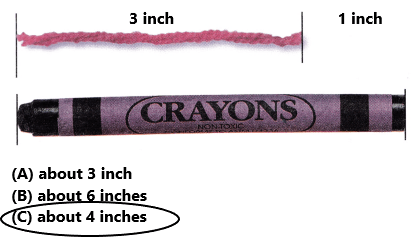Texas Go Math Grade 2 Lesson 16.1 Answer Key Measure with Inch Models

Refer to our Texas Go Math Grade 2 Answer Key Pdf to score good marks in the exams. Test yourself by practicing the problems from Texas Go Math Grade 2 Lesson 16.1 Answer Key Measure with Inch Models.

Texas Go Math Grade 2 Lesson 16.1 Answer Key Measure with Inch Models

Explore

Use color tiles to measure the length.___________ color tiles___________ color tiles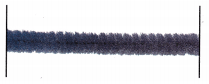___________ color tilesExplanation:
The first ribbon is about 3 color tiles long
The second ribbon is about 4 color tiles long
The third ribbon is about 2 color tiles long.

FOR THE TEACHER • Read the following problem. Manny wants to know the lengths of his three chenille sticks. How can Manny measure the sticks?

Math Talk
Mathematical Processes

Describe how to use color tiles to measure the length an object.
To measure length of an object using color tiles, place color tiles along the length of the object without giving gaps. Then count the number of tiles. The length of the object will be equal to the number of tiles.

Model and Draw

A color tile is about 1 inch long.
About how many inches long is this string?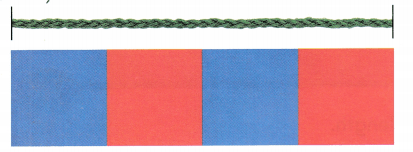Count the color tiles to measure the length of the string in inches.
The string is 4 color tiles long.
So, the string is about __________ inches long.
I counted the color tiles to measure the length of the string in inches.
The string is 4 color tiles long.
So, the string is about 4 inches long.

Share and Show

Use color tiles. Measure the length of the object in inches.

Question 1.Explanation:
I counted the color tiles to measure the length of the string in inches.
The string is 3 color tiles long.
So, the string is about 3 inches long.

Question 2.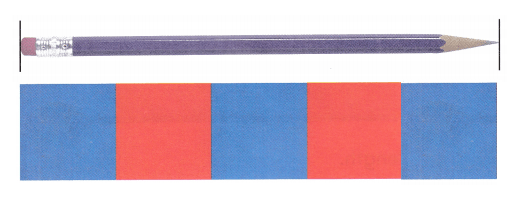Explanation:
I counted the color tiles to measure the length of the string in inches.
The string is 5 color tiles long.
So, the string is about 5 inches long.

Question 3.Explanation:
I counted the color tiles to measure the length of the string in inches.
The string is 2 color tiles long.
So, the string is about 2 inches long.

Question 4.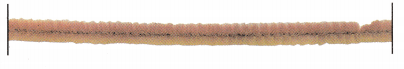Explanation:
I counted the color tiles to measure the length of the string in inches.
The string is 4 color tiles long.
So, the string is about 4 inches long.

Problem Solving

Use color tiles. Measure the length of the object in inches.

Question 5.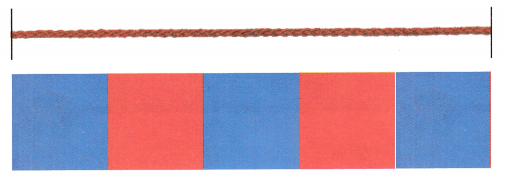Explanation:
I counted the color tiles to measure the length of the string in inches.
The string is 5 color tiles long.
So, the string is about 5 inches long.

Question 6.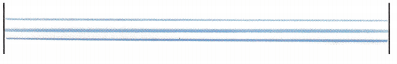Explanation:
I counted the color tiles to measure the length of the string in inches.
The string is 4 color tiles long.
So, the string is about 4 inches long.

Question 7.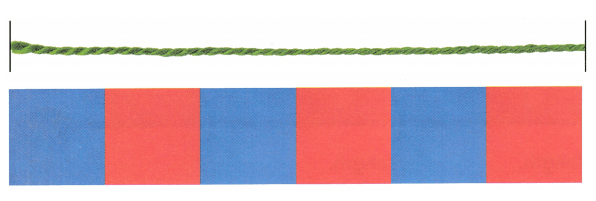Explanation:
I counted the color tiles to measure the length of the string in inches.
The string is 6 color tiles long.
So, the string is about 6 inches long.

Solve.

Question 8.
H.O.T. Multi-Step Blue paper chains are 8 inches long. Red paper chains are 6 inches long. How many are needed to have 22 inches of paper chains?_________ blue paper chains
_________ red paper chains
2 blue paper chains
1 red paper chains

Explanation:
Blue paper chains are 8 inches long. Red paper chains are 6 inches long
8+8+6=24
To make 22 we need 2 blue paper chains and 1 red paper chains
Therefore to have 22 inches of paper chains we need 2 Blue paper chains and 1 Red paper chain.

Question 9.
H.O.T. Liza has a piece of ribbon that is 12 inches long. She needs to cut it into pieces that are each 4 inches long. How many pieces can she make?
___________ pieces
3 pieces

Explanation:
Liza has a piece of ribbon that is 12 inches long. She needs to cut it into pieces that are each 4 inches long
To find the number of pieces of ribbon divide 12 by 4
12/4=3
Therefore, Liza can make 3 pieces of ribbon.

Question 10.
Use color tiles to measure. Which is the best choice for the length of the crayon?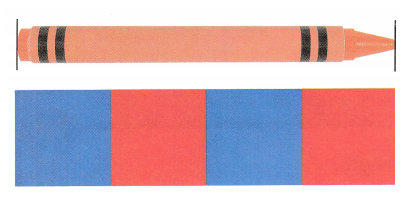Explanation:
I counted the color tiles to measure the length of the crayon in inches.
The crayon is 4 color tiles long.
So, the crayon is about 4 inches long.

Question 11.
Analyze The ribbon is about 5 inches long. Which is the best choice for the length of the yarn?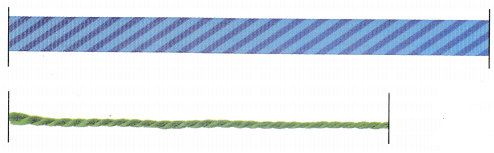Explanation:
I counted the color tiles to measure the length of the yarn in inches.
The yarn is 4 color tiles long.
So, the yarn is about 4 inches long.

Question 12.
Texas Test Prep Jeremy used color tiles to measure the yarn. Which is the best choice for the length of the yarn?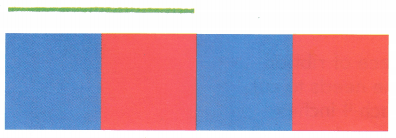Explanation:
I counted the color tiles to measure the length of the yarn in inches.
The yarn is 2 color tiles long.
So, the yarn is about 2 inches long.

TAKE HOME ACTIVITY • Have your child use several of the some small item (such as paper clips), to measure the lengths of some objects at home.

Texas Go Math Grade 2 Lesson 16.1 Homework and Practice Answer Key

Each tile is 1 inch long. What is the length of the object in inches.

Question 1.Explanation:
In the above image we can observe each tile represents 1 inch long. I counted the color tiles to measure the length of the object in inches. There are three tiles. So, the length of the object is about 3 inches long.
Question 2.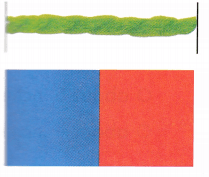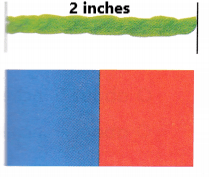Explanation:
In the above image we can observe each tile represents 1 inch long. I counted the color tiles to measure the length of the object in inches. There are two tiles. So, the length of the object is about 2 inches long.

Problem Solving

Question 3.
Multi-Step Blue paper chains are 4 inches long. Red paper chains are 3 inches long. How many are needed to have 10 inches of paper chains?
_____________ blue paper chain
_____________ red paper chain
4 inches + 3 inches = 7 inches
10 inches – 7 inches = 3 inches
1 blue paper chain
2 red paper chain
Explanation:
Lets take one blue chain and one red chain. So, the total inches will be 7 (4 + 3= 7).
In order to have 10 inches of paper chains, we still need 3 inches (10 – 7 = 3) that is another red paper chain.
So, finally we need 1 blue paper chains and two red paper chains.

Lesson Check

Question 4.
The yarn is about 4 inches long. Which is the best choice for the length of the pen?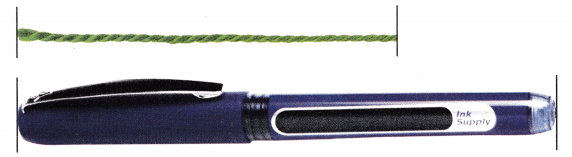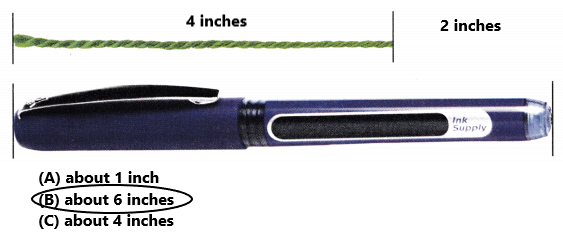Option B is correct.
Explanation:
The yarn is about 4 inches long. The remaining length of the yarn is about 2 inches long. Add 4 inches with 2 inches the sum is 6 inches. The length of the pen is about 6 inches long. So, draw a circle for option B.

Question 5.
The ribbon is about 4 inches long. Which is the best choice for the length of the yarn?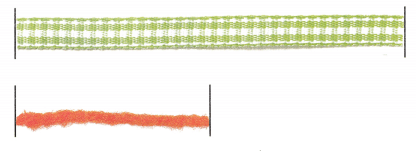Option A is correct.
Explanation:
The ribbon is about 4 inches long as we can observe in the above image. The length of the yarn is half of the ribbon length. The length of the yarn is about 2 inch long. So, draw a circle for option A.

Question 6.
The yarn is about 3 inches long. Which is the best choice for the length of the crayon?